## Symmetric Matrix

A square matrix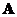is symmetric if its transpose is equal to itself, that is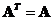Symmetric matrix is important in many applications because of its properties. Examples of well known symmetric matrices are correlation matrix, covariance matrix and distance matrix.

You can easily create symmetric matrix either by

1. Multiplying a matrix by its transpose: if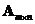is a rectangular matrix, then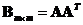and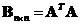are symmetric matrices.
2. Adding a matrix by its transpose: if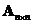is a square matrix, then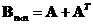is a symmetric matrix.

The interactive program below is designed to answers the question whether the given input matrix is a symmetric matrix. When you click Random Example button, it will create random input matrix to provide you with many examples of symmetric and non-symmetric matrices. You may also want to try to type your own input matrix to test whether it is a symmetric matrix.

## Properties

Some important properties of symmetric matrix are

• Symmetric matrix is always a square matrix
• Ifis a symmetric matrix order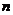with real entries then
o The transpose matrixis also a symmetric matrix
o Scalar multiple of the matrix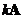is also a symmetric matrix
o The inverse matrix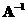is also a symmetric matrix, if it is invertible.
o The inverse of the transpose matrix is equal to the matrix inverse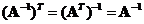o A half of the summation to its transpose produces the matrix itself,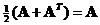o Subtraction to its transpose produces null matrix,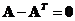o Symmetric matrixhas linearly independent Eigen vectors.
o The Eigen values of symmetric matrixare all real numbers (no complex numbers).
o If all eigenvalues of symmetric matrixare distinct (no multiple Eigen values), then matrix A can be transformed into diagonal matrix
o Eigenvectors of distinct eigenvalues are orthogonal.
o The number of non-zero eigenvalues is equal to its rank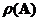.
o There is an orthogonal matrix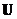that diagonalizes symmetric matrixby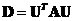(spectral decomposition).
• Ifand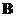are symmetric matrices of the same size, then
o The summation of the matrix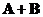is also a symmetric matrix
o The subtraction of the matrix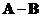is also a symmetric matrix, if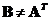• Letbe any rectangular matrix size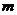bysuch that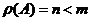, then we can form symmetric matrix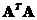and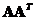. The non-zero eigenvalues ofandare equal. The rank of both matrices are equal,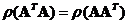.
• Hermitian matrix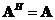is a symmetric matrix with entries of complex number (across the diagonal, the entries are complex conjugate).
• Both Hermitian and Unitary matrix (including symmetric and orthogonal matrix) are called normal matrix because the Eigen vectors form orthonormal set.